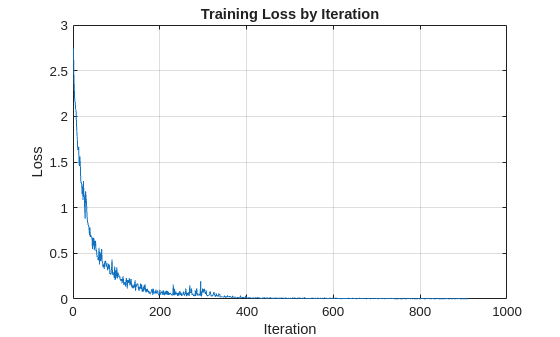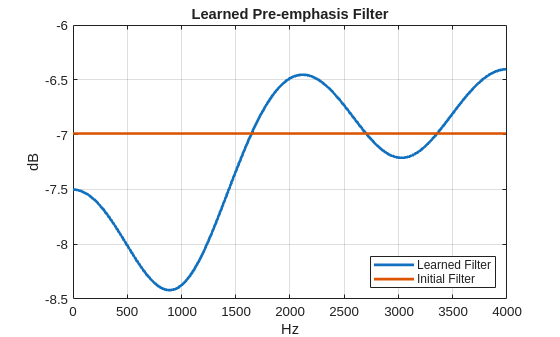# Learn Pre-Emphasis Filter Using Deep Learning

This example shows how to use a convolutional deep network to learn a pre-emphasis filter for speech recognition. The example uses a learnable short-time Fourier transform (STFT) layer to obtain a time-frequency representation suitable for use with 2-D convolutional layers. The use of a learnable STFT enables a gradient-based optimization of the pre-emphasis filter weights.

### Data

Clone or download the Free Spoken Digit Dataset (FSDD), available at https://github.com/Jakobovski/free-spoken-digit-dataset. FSDD is an open data set, which means that it can grow over time. This example uses the version committed on 08/20/2020 which consists of 3000 recordings of the English digits 0 through 9 obtained from six speakers. The data is sampled at 8000 Hz.

This example assumes that you have downloaded the data into the folder corresponding to the value of `tempdir` in MATLAB. If you use a different folder, substitute that folder name for `tempdir` in the following code. Use audioDatastore to manage data access and ensure random division of data into training and test sets.

```pathToRecordingsFolder = fullfile(tempdir,'free-spoken-digit-dataset','recordings'); ads = audioDatastore(pathToRecordingsFolder);```

The helper function `helpergenLabels` creates a categorical array of labels from the FSDD files. The source code for `helpergenLabels` is listed in the appendix. List the classes and the number of examples in each class. It may take a few minutes to generate all the labels for this dataset.

```ads.Labels = helpergenLabels(ads); summary(ads.Labels)```
``` 0 300 1 300 2 300 3 300 4 300 5 300 6 300 7 300 8 300 9 300 ```

Split the FSDD into training and test sets maintaining equal class proportions in each subset. For reproducible results, set the random number generator to its default value. Eighty percent, or 2400 recordings, are used for training. The remaining 600 recordings, 20% of the total, are held out for testing. Shuffle the files in the datastore once before creating the training and test sets.

```rng default; ads = shuffle(ads); [adsTrain,adsTest] = splitEachLabel(ads,0.8,0.2);```

The recordings in FSDD are not equal in length. Use a transform so that each read from the datastore is padded or truncated to 8192 samples. The data are additionally cast to single-precision and a z-score normalization is applied.

```transTrain = transform(adsTrain,@(x,info)helperReadData(x,info),'IncludeInfo',true); transTest = transform(adsTest,@(x,info)helperReadData(x,info),'IncludeInfo',true);```

### Deep Convolutional Neural Network (DCNN) Architecture

This example uses a custom training loop with the following deep convolutional network.

```numF = 12; dropoutProb = 0.2; layers = [ sequenceInputLayer(1,'Name','input','MinLength',8192,... 'Normalization',"none") convolution1dLayer(5,1,"name","pre-emphasis-filter",... "WeightsInitializer",@(sz)kronDelta(sz),"BiasLearnRateFactor",0) stftLayer('Window',hamming(1280),'OverlapLength',900,... 'OutputMode','spatial','Name','STFT') convolution2dLayer(5,numF,'Padding','same') batchNormalizationLayer reluLayer maxPooling2dLayer(3,'Stride',2,'Padding','same') convolution2dLayer(3,2*numF,'Padding','same') batchNormalizationLayer reluLayer maxPooling2dLayer(3,'Stride',2,'Padding','same') convolution2dLayer(3,4*numF,'Padding','same') batchNormalizationLayer reluLayer maxPooling2dLayer(3,'Stride',2,'Padding','same') convolution2dLayer(3,4*numF,'Padding','same') batchNormalizationLayer reluLayer convolution2dLayer(3,4*numF,'Padding','same') batchNormalizationLayer reluLayer maxPooling2dLayer(2) dropoutLayer(dropoutProb) fullyConnectedLayer(numel(categories(ads.Labels))) softmaxLayer ]; dlnet = dlnetwork(layers);```

The sequence input layer is followed by a 1-D convolution layer consisting of a single filter with 5 coefficients. This is a finite impulse response filter. Convolutional layers in deep learning networks by default implement an affine operation on the input features. To obtain a strictly linear (filtering) operation, use the default `'BiasInitializer'` which is `'zeros'` and set the bias learn rate factor of the layer to 0. This means that the bias is initialized to 0 and never changes during training. The network uses a custom initialization of the filter weights to be a scaled Kronecker delta sequence. This is an allpass filter, which performs no filtering of the input. The code for the allpass filter weight initializer is shown here.

```function delta = kronDelta(sz) % This function is only for use in the "Learn Pre-Emphasis Filter using % Deep Learning" example. It may change or be removed in a % future release. L = sz(1); delta = zeros(L,sz(2),sz(3),'single'); delta(1) = 1/sqrt(L); end ```

`stftLayer` takes the filtered batch of input signals and obtains their magnitude STFTs. The magnitude STFT is a 2-D representation of the signal, which is amenable to use in 2-D convolutional networks.

While the weights of the STFT are not changed here during training, the layer supports backpropagation, which enables the filter coefficients in the "pre-emphasis-filter" layer to be learned.

### Network Training

Set the training options for the custom training loop. Use 25 epochs with a minibatch size of 128. Set the initial learn rate to 0.001.

```NumEpochs = 25; miniBatchSize = 128; learnRate = 0.001;```

In the custom training loop, use a `minibatchqueue` object. The `processSpeechMB` function reads in a minibatch and applies a one-hot encoding scheme to the labels.

```mbqTrain = minibatchqueue(transTrain, 2,... 'MiniBatchSize',miniBatchSize,... 'MiniBatchFormat', {'CBT', 'CB'}, ... 'MiniBatchFcn', @processSpeechMB);```

Train the network and plot the loss for each iteration. Use an Adam optimizer to update the network learnable parameters. To plot the loss as training progress, set the value of `progress` in the following code to "training-progress".

```progress = "final-loss"; if progress == "training-progress" figure lineLossTrain = animatedline; ylim([0 inf]) xlabel("Iteration") ylabel("Loss") grid on end % Initialize some training loop variables. trailingAvg = []; trailingAvgSq = []; iteration = 0; lossByIteration = 0; % Loop over epochs. Time the epochs. start = tic; for epoch = 1:NumEpochs reset(mbqTrain) shuffle(mbqTrain) % Loop over mini-batches. while hasdata(mbqTrain) iteration = iteration + 1; % Get the next minibatch and one-hot coded targets [dlX,Y] = next(mbqTrain); % Evaluate the model gradients and loss [gradients, loss, state] = dlfeval(@modelGradSTFT,dlnet,dlX,Y); if progress == "final-loss" lossByIteration(iteration) = loss; end % Update the network state dlnet.State = state; % Update the network parameters using an Adam optimizer. [dlnet,trailingAvg,trailingAvgSq] = adamupdate(... dlnet, gradients, trailingAvg, trailingAvgSq, iteration, learnRate); % Display the training progress. D = duration(0,0,toc(start),'Format','hh:mm:ss'); if progress == "training-progress" addpoints(lineLossTrain,iteration,loss) title("Epoch: " + epoch + ", Elapsed: " + string(D)) end end disp("Training loss after epoch " + epoch + ": " + loss); end```
```Training loss after epoch 1: 0.78417 Training loss after epoch 2: 0.33268 Training loss after epoch 3: 0.28916 Training loss after epoch 4: 0.1111 Training loss after epoch 5: 0.14384 Training loss after epoch 6: 0.030357 Training loss after epoch 7: 0.020752 Training loss after epoch 8: 0.04275 Training loss after epoch 9: 0.022452 Training loss after epoch 10: 0.060283 Training loss after epoch 11: 0.013778 Training loss after epoch 12: 0.018867 Training loss after epoch 13: 0.02545 Training loss after epoch 14: 0.0073273 Training loss after epoch 15: 0.0020516 Training loss after epoch 16: 0.0058642 Training loss after epoch 17: 0.0021439 Training loss after epoch 18: 0.016893 Training loss after epoch 19: 0.017999 Training loss after epoch 20: 0.014562 Training loss after epoch 21: 0.007233 Training loss after epoch 22: 0.00099405 Training loss after epoch 23: 0.0053691 Training loss after epoch 24: 0.00087165 Training loss after epoch 25: 0.00034627 ```
```if progress == "final-loss" plot(1:iteration,lossByIteration) grid on title('Training Loss by Iteration') xlabel("Iteration") ylabel("Loss") end```Test the trained network on the held-out test set. Use a `minibatchqueue` object with a minibatch size of 32.

```miniBatchSize = 32; mbqTest = minibatchqueue(transTest, 2,... 'MiniBatchSize',miniBatchSize,... 'MiniBatchFormat', {'CBT', 'CB'}, ... 'MiniBatchFcn', @processSpeechMB);```

Loop over the test set and predict the class labels for each minibatch.

```numObservations = numel(adsTest.Files); classes = string(unique(adsTest.Labels)); predictions = []; % Loop over mini-batches. while hasdata(mbqTest) % Read mini-batch of data. dlX = next(mbqTest); % Make predictions on the minibatch dlYPred = predict(dlnet,dlX); % Determine corresponding classes. predBatch = onehotdecode(dlYPred,classes,1); predictions = [predictions predBatch]; end```

Evaluate the classification accuracy on the 600 examples in the held-out test set.

`accuracy = mean(predictions' == categorical(adsTest.Labels))`
```accuracy = 0.9783 ```

Test performance is approximately 98%. You can comment out the 1-D convolution layer and retrain the network without the pre-emphasis filter. The test performance without the pre-emphasis filter is also excellent at approximately 96%, but the use of the pre-emphasis filter makes a small improvement. It is noteworthy, that while the use of the learned pre-emphasis filter has only improved the test accuracy slightly, this was achieved by adding only 5 learnable parameters to the network.

To examine the learned pre-emphasis filter, extract the weights of the 1-D convolutional layer. Plot the frequency response. Recall that the sampling frequency of the data is 8 kHz. Because we initialized the filter to a scaled Kronecker delta sequence (allpass filter), we can easily compare the frequency response of the initialized filter with the learned response.

```FIRFilter = dlnet.Layers(2).Weights; [H,W] = freqz(FIRFilter,1,[],8000); delta = kronDelta([5 1 1]); Hinit = freqz(delta,1,[],4000); plot(W,20*log10(abs([H Hinit])),'linewidth',2) grid on xlabel('Hz') ylabel('dB') legend('Learned Filter','Initial Filter','Location','SouthEast') title('Learned Pre-emphasis Filter')```This example showed how to learn a pre-emphasis filter as a preprocessing step in a 2-D convolutional network based on short-time Fourier transforms of the signals. The ability of `stftLayer` to support backpropagation enabled gradient-based optimization of the filter weights inside the deep network. While this resulted in only a small improvement in the performance of the network on the test set, it achieved this improvement with a trivial increase in the number of learnable parameters.

### Appendix: Helper Functions

```function Labels = helpergenLabels(ads) % This function is only for use in the "Learn Pre-Emphasis Filter using % Deep Learning" example. It may change or be removed in a % future release. tmp = cell(numel(ads.Files),1); expression = "[0-9]+_"; for nf = 1:numel(ads.Files) idx = regexp(ads.Files{nf},expression); tmp{nf} = ads.Files{nf}(idx); end Labels = categorical(tmp); end function [out,info] = helperReadData(x,info) % This function is only for use in the "Learn Pre-Emphasis Filter using % Deep Learning" example. It may change or be removed in a % future release. N = numel(x); x = single(x); if N > 8192 x = x(1:8192); elseif N < 8192 pad = 8192-N; prepad = floor(pad/2); postpad = ceil(pad/2); x = [zeros(prepad,1) ; x ; zeros(postpad,1)]; end x = (x-mean(x))./std(x); x = x(:)'; out = {x,info.Label}; end function [dlX,dlY] = processSpeechMB(Xcell,Ycell) % This function is only for use in the "Learn Pre-Emphasis Filter using % Deep Learning" example. It may change or be removed in a % future release. Xcell = cellfun(@(x)reshape(x,1,1,[]),Xcell,'uni',false); dlX = cat(2,Xcell{:}); dlY = cat(2,Ycell{:}); dlY = onehotencode(dlY,1); end function [grads, loss, state] = modelGradSTFT(net, X, T) % This function is only for use in the "Learn Pre-Emphasis Filter using % Deep Learning" example. It may change or be removed in a % future release. [y, state] = net.forward(X); loss = crossentropy(y, T); grads = dlgradient(loss,net.Learnables); loss = double(gather(extractdata(loss))); end ```